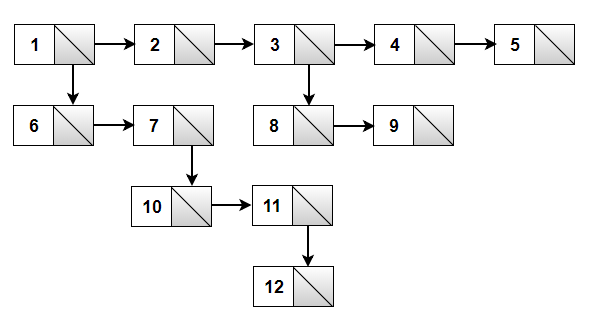### C++ program to Convert a multi level linked list to a singly linked list

Introduction:

Let us try to understand it with an example. The multilevel linked list is similar to the simple linked list, except that it has one extra field that points to that node’s child. The child may point to a separate list altogether, which may have children of its own.

For example, consider the following multilevel linked list:We should convert it into list 1—>2—>3—>4—>5—>6—>7—>8—>9—>10—>11—>12—>null.

The idea is to use the queue data structure to solve this problem. We start by traversing the list horizontally from the head node using the next pointer, and whenever a node with a child is found, insert the child node into a queue. If the end of the list is reached, dequeue the front node, set it as the next node of the last encountered node, and repeat the entire process till the queue becomes empty.

Program:

#include <iostream>

#include <vector>

#include <queue>

using namespace std;

struct Node

{

int data;

Node *next, *child;

};

// Helper function to create a new node with the given data and

// pushes it onto the list's front

{

Node* newNode = new Node;

newNode->data = data;

newNode->child = nullptr;

}

// Helper function to create a linked list with elements of a given vector

Node* createHorizontalList(vector<int> const &input)

{

for (int i = input.size() - 1; i >= 0; i--) {

}

}

// Function to convert a multilevel linked list into a singly linked list

{

queue<Node*> q;

// process all nodes

while (curr)

{

// last node is reached

if (curr->next == nullptr)

{

// dequeue the front node and set it as the next node of the current node

curr->next = q.front();

q.pop();

}

// if the current node has a child

if (curr->child) {

q.push(curr->child);

}

curr = curr->next;

}

}

// Helper function to print a given linked list

{

while (ptr)

{

cout << ptr->data << " —> ";

ptr = ptr->next;

}

cout << "nullptr" << endl;

}

int main()

{

// create a multilevel linked list

Node* head = createHorizontalList({1, 2, 3, 4, 5});

return 0;

}

Output:

1 —> 2 —> 3 —> 4 —> 5 —> 6 —> 7 —> 8 —> 9 —> 10 —> 11 —> 12 —> NULL#### More Articles of Shaik Aftab Ahmed:

Name Views Likes
C++ Program to Find the Frequency of Odd & Even Numbers in the given Matrix 311 1
C++ program to Sort a Linked List Using Merge Sort 282 1
C++ Program to Implement a Linked List representation of a Binary tree 268 1
C++ Program to Check for balanced parentheses in an expression 182 1
C++ Program to Perform Inorder, Preorder, Postorder traversals in a binary tree. 229 1
C++ program to print Indian flag 268 1
C++ program to Convert a multi level linked list to a singly linked list 182 1
C++ program to print right view of a Binary tree using queue 197 1
C++ Program to implement Huffman Coding Compression Algorithm 822 1
C++ Program to Create a Height Balanced Binary Tree 213 1
C++ program to implement Prims algorithm 310 1
C++ Program for BFS Traversal 217 1
C++ Progam to Evaluate a Prefix Expression 205 1
C++ Program to Implement Queue using Linked List 210 1
C++ implementation of Stack using Linked list 227 1
C++ program to find the intersection point of two linked lists 256 1
C++ program to count the inversions in the given array 236 1
C++ program to perform D.N.F sort 276 1
C++ program to print all possible subsets of a String 249 1
C++ program to count the number of ones in a binary representation of a number 256 1
C++ program to print all possible subsets of a set 280 1
C++ program to find the largest word in a String 241 1
C++ Program to print a matrix in Spiral order 278 1
C++ program to convert from Binary to Decimal, Octal to Decimal, Hexadecimal to Decimal 266 1
C limits library 298 1
Program to add two Binary numbers 269 1